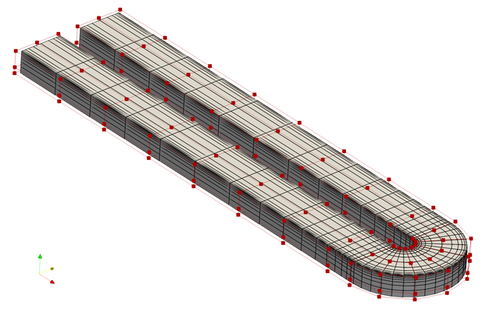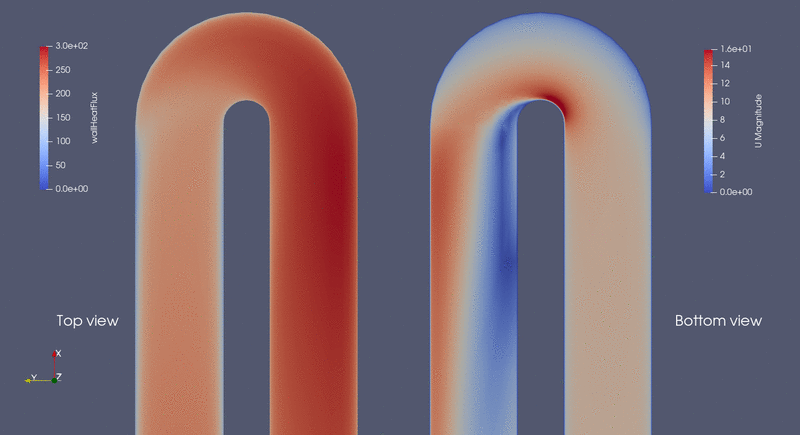The following is a heat transfer optimization case for the von Karman U bend channel, representative of a internal cooling section in turbines.

```Case: Heat transfer optimization for U bend cooling channels
Geometry: von Karman U bend channel
Objective function: Combined wall heat flux and total pressure loss
Design variables: 114 FFD points moving in the x, y, and z directions
Constraints: Symmetry constraint (total number: 38)
Mach number: 0.02
Reynolds number: 4.2e4
Mesh cells: 4.8 K
Solver: DASimpleTFoam
```Fig. 1. Mesh and FFD points for the U bend channel case

In this case, we set up a combined objective function of wall heat flux and total pressure loss. This is done by setting three parts for the objFunc. part1 and part2 are the total pressure at the inlet and outlet, respectively. part3 is the wall heat flux. The weights between the heat flux and total pressure loss is 0.5.

```"objFunc": {
"obj": {
"part1": {
"type": "totalPressure",
"source": "patchToFace",
"patches": ["inlet"],
"scale": 1.0 / CPL0 * CPL_weight,
},
"part2": {
"type": "totalPressure",
"source": "patchToFace",
"patches": ["outlet"],
"scale": -1.0 / CPL0 * CPL_weight,
},
"part3": {
"type": "wallHeatFlux",
"source": "patchToFace",
"patches": ["ubendup"],
"scale": 1.0 / HFL0 * HFL_weight,
}
},
},
```

To run this case, first download tutorials and untar it. Then go to tutorials-main/UBend_Channel and run the “preProcessing.sh” script to generate the mesh:

```./preProcessing.sh
```

Then, use the following command to run the optimization with 4 CPU cores:

```mpirun -np 4 python runScript.py 2>&1 | tee logOpt.txt
```

This case ran for 18 iterations. The heat flux remained the same while the total pressure loss reduced by 53.4%.Fig. 2. Evolution of wall heat flux and velocity during the optimization